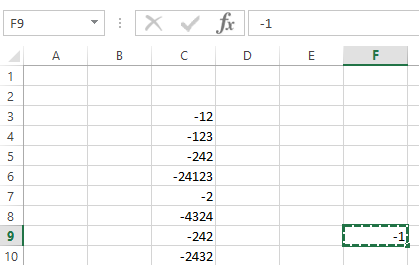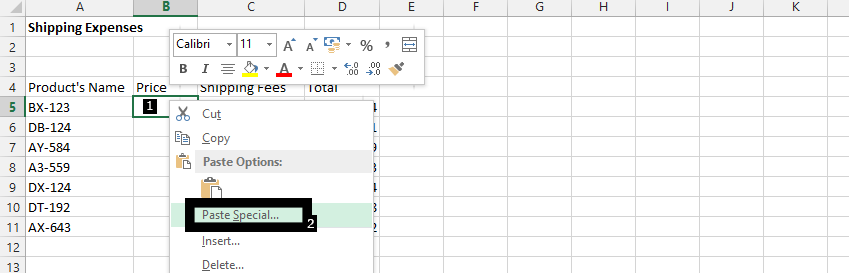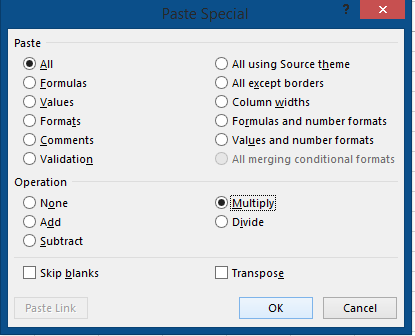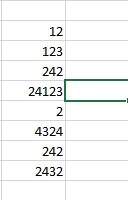#### How to convert negative number to positive?

The steps below shows how to convert a negative number to a positive number.

## Data preparation

Write -1 in a blank cell, copy it and then select the negative numbers.

Note: If a negative number are not adjacent, select the first negative number and then hold Ctrl key, and select the other negative numbers.After you have selected all the negative number, right click, a drop down menu will appear from which you have to select “Paste Special”.## Using the Paste Special dialog box

A new drop down menu will appear. Select “Paste Special” option at the bottom of the drop down menu then select “Multiply” option from the operation. Click ok.All the negative number selected are now positive.## Using the ABS function

The ABS function in Excel returns the absolute value of a number, which is the positive value of the number, regardless of whether it’s positive or negative. To use the ABS function to convert a negative number to positive, simply type the following formula into a cell and press Enter:

`=ABS(cell reference)`

Where cell reference is the cell containing the negative number that you want to convert. For example, if the negative number is in cell A1, the formula would be:

`=ABS(A1)`

This will return the positive value of the number in cell A1.